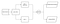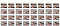# Introduction and background

The goal of this article is to explain the logic flow behind the fast.ai’s Datablock API, and how to write the functions to enable the use of this API with video data.

Recently while working on a project to detect whether or not a page in a book has been turned using video, the first thing that needed to be solved was how to load video data into a neural network. The network architecture is going to be based on this paper, with the goal of classifying a video between two classes, or actions. The framework I chose to use was fast.ai, which is a library based on PyTorch. The built in high-level API only natively supports loading image data.

While there is no plug and play solution, we can utilize the existing mid-level API to load and format our data into what we need. Simply put, video data can be represented as two parts, a the visual stream of data, which can be expressed as a collection of frames, and the audio stream of data, which is not relevant to this project. So in essence, the goal of this task was to figure out a way to feed in a collection of images, or frames, into a neural network.

# Brief Overview of DataBlock API

There is a great tutorial here to understand how to build a DataBlock from scratch. In this post we will focus on identifying the logic flow between the different parts of a DataBlock. If we take a look at this code sample here:

`dblock = DataBlock(    blocks      = (ImageSequenceBlock, CategoryBlock),    get_items   = get_items,     get_x       = get_x,     get_y       = get_y,     splitter    = splitter,    batch_tfms  = aug_transforms())`

So let’s start by defining the different parameters for DataBlock.

• blocks — Blocks represent the final format of the data being sent into. In this case the first block represents the video or sequence of images being sent in, and the second block is the accompanying label to the data.
• get_items — This function is called initially when accessing the DataBlock. This function defines where to get the data from, whether it be from a local source or cloud.
• get_x — If the return of your get_items can be used as your data source, then this function is optional. Otherwise, you can utilize this function to do extra processing on the return from get_items to get your X or variable data.
• get_y — This function is used to generate the label for the data. In this example, the data from this function ultimately ends up being used in the CategoryBlock under blocks.
• splitter — This function determines how your data is spit between training and test sets.
• batch_tfms — This is a collection of functions that will be used to augment your data. This is especially important when working with image or visual data to train your models.

A basic overview without transformations or the splitter looks like this:Logic flow from Data Source to DataBlocks.

Something to note in this diagram is that the splitter can represent nodes for the training and test set.

The next section will cover how we used these existing parameters to load and augment our data, parameter by parameter.

# Implementation Details

To begin we must first define what we want our data to look like before getting sent to the model.

Initially we can define the ImageSequence class, which ultimately returns a tuple of image files.

`class ImageSequence(tuple):     def create(image_files):          return tuple(PILImage.create(f) for f in image_files)`

Nothing fancy going on here, all this object expects is a list of path objects or paths to the image files for the current batch.

Now that we a representation of what the data will look like, we can build a layer on top of that to represent the data as a datablock for fast.ai.

`def ImageSequenceBlock():     return TransformBlock(type_tfms = ImageSequence.create)`

What we are doing here is taking the built-in TransformBlock and supplying a function to define how the data should end up.

For the label we can use the built-in CategoryBlock.

Now we must define the class that will load. In our implementation we will utilize get_items to gather the file names to be passed to the ImageSequence class we defined above.

`class SequenceGetItems():     def __init__(self, dataset_path):          self.dataset_path = dataset_path     def __call__(self, source):        # get file names of all files        fns = get_image_files(self.dataset_path)        # intialize vid_index dictionary and video frames list        vid_index = {}        vids = []        # loop through file names        for fn in fns:            # get label and video id            split = str(fn).split("\\")            label = split[-3]            vid_id = split[-2]                        # if video is not flip, add extension to id to differentiate for videos with same title            if label == 'notflip':                vid_id = vid_id + 'a'                        # add video to index dict if not exists yet            if vid_id not in vid_index.keys():                vid_index[vid_id] = len(vids)                vids.append([fn])            else:                # if exists, add current frame to existing list of frames                index = vid_index[vid_id]                existing_frames = vids[index]                existing_frames.append(fn)                vids[index] = existing_frames        # sort all vides by frame name        for i in range(len(vids)):            vids[i] = sorted(vids[i], key=lambda v: int(v.stem))                return vids`

What the class above is doing is initializing the path for the dataset when initializing the class, and returns a list of lists. Each sub list represents a list of path objects for each frame in a single video. This data then gets passed on to get_x and get_y.

get_x and get_y can be defined as lambda functions:

`get_x = lambda v: random_crop_video(v, min_length = 16)get_y = lambda v: str(v).split('\\')[-3]`

For get_y, we can extract the label from the filenames of the image files. We just take the first one of the batch and because the label is inside the path name we can get it that way. This method can be modified based on the use case, but remember that the data available for this step must be provided by get_items.

For get_x we take a random crop of the video frames to fit the minimum length. Remember, training the model will require all of our data to be in the same format. In this case we use 16.

`def random_crop_video(video, length):    """    get a random crop of video frames sequentially    if frames are under max, multiply some frames at random    """    frame_difference = min_length - len(vid_frames)    if frame_difference > 0:        for i in range(0, frame_difference):            random_index = round(len(vid_frames) * np.random.rand())            random_frame = vid_frames[random_index]            pre = vid_frames[0:random_index]            pre.append(random_frame)            post = vid_frames[random_index:]            vid_frames = pre + post    elif frame_difference < 0:        random_start = round(np.random.rand() * frame_difference *     -1)        vid_frames = vid_frames[random_start:(random_start +  min_length)]    return vid_frames`

In the above function a couple things are happening. First we must compare the existing frame count with the frame count we want. If we have more than enough, then we can choose a random starting index and take a crop from there.
In the case where we don’t have enough frames, I ended up implementing a strategy to copy random frames and double them to fill the space. Other strategies like extending the beginning or end of the video can be utilized and will have to be tested with the model performance.

Next we use the splitter to determine if the data is being used in the test set or the training set. To split our data we defined this splitter:

`splitter = FuncSplitter(lambda o: Path(o.parent.parent.parent.name =='test')`

Since we had the set the data belonged to in the path we were able to utilize it. There are also other built-in splitters like randomsplitter() or grandparentsplitter().

`splitter = FuncSplitter(lambda o: Path(o).parent.parent.parent.name == 'test')get_x = lambda v: random_crop_video(v, min_length = 16)get_y = lambda v: str(v).split('\\')[-3]dblock = DataBlock(    blocks      = (ImageSequenceBlock, CategoryBlock),    get_items   =  SequenceGetItems(PATH_TO_DATASET),     get_x       =  get_x,     get_y       =  get_y,     splitter    =  splitter)`

With the code above we have now generated our DataBlock object. We can use this to handle our data. This is also where we would attach any transformations to the data. Since we are essentially using 3D data, the built-in transforms do not support this natively. Stay tuned for my next post which will cover how to do just that!

Congrats, if you have been following till now you have successfully defined your custom DataBlock object. Now what? Well now you can use the dataloaders attribute from the DataBlock to load your data. Like so:

`dls = dblock.dataloaders(os.path.join('data', 'turning'),                          bs=4,                         create_batch=create_batch)`

You will notice that there is a function here create_batch. This function will act as a way to do final processing on our data before it gets sent to the model. Currently the DataBlock is returning essentially a list of images and a label. What the model is expecting is a PyTorch tensor.

`def create_batch(data):    xs, ys = [], []    for d in data:        xs.append(d)        ys.append(d)    xs = torch.cat([TensorImage(torch.cat([im[None] for im in x],   dim=0))[None] for x in xs], dim=0)    ys = torch.cat([y[None] for y in ys], dim=0)    return TensorImage(xs), TensorCategory(ys)`

This is what the code above is doing:
1. Appending the batch of images and label into temporary lists, xs holding the former.
2. With the xs list, we can manipulate it by first concatenating the image tensors into a tensor with a new dimension, then bundling that up together as an image tensor.
3. With the ys list, we just transform it into a tensor
4. We return xs and ys wrapped in built-in fast.ai wrappers for TensorImage and TensorCategory.

For reference, if you have a batch of 8 photos per sequence, that are 256x256, the shape of a tensor for a single batch would be [1, 8, 3, 256, 256].

It is always helpful to displaying your batches so you know what you are working with. You can use the code below to display batches for your data.

`def show_sequence_batch(dls, max_n=4):    """    shows max_n batches of data from the dataloader    """    xb, yb = dls.one_batch()    fig, axes = plt.subplots(ncols=16, nrows=max_n, figsize=(35,6), dpi=120)    for i in range(max_n):        xs, ys = xb[i], yb[i]        for j, x in enumerate(xs):            axes[i,j].imshow(x.permute(1,2,0).cpu().numpy())            axes[i,j].set_title(ys.item())            axes[i,j].axis('off')show_sequence_batch(dls, 4)`

In show_sequence_batch what is happening is that we take a single batch from our newly created dataloader and setting up some subplots using matplotlib to place our images. Next we iterate for however many rows we want to display, and iterate througha batch of images. For each image we must first change the change the existing tensor shape of the image to match the parameters for the .imshow function, make sure the tensor is located on the cpu memory, and change it to a numpy array.

Here is a sample for a few batches from the dataset I was using:0 represents a page being flipped in the video, 1 represents a page not being flipped

# Conclusion

In this post we covered the basic logic and flow of data behind the DataBlock API. There are other things you can do like adding transforms to augment the data, which will be covered in a future post.

Learn something new? Have a suggestions? Let’s connect on LinkedIn

## More from Jo Vincent Ruiz

Machine Learning Engineer